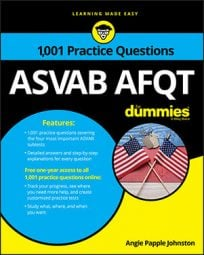##### ASVAB AFQTWhen you encounter logarithm problems on the Mathematics Knowledge subtest on the ASVAB, you can use the log rule to solve them. The following practice questions show you how.

## Practice questions

1. Solve: ln(42x)

A. 2xln(2) B. 4xln(2) C. log24ln2 D. ln(22)

2. Solve: log464 = x

A. 4 B. 3 C. –4 D. 7

1. The correct answer is Choice (B).

Simplify and apply the log rule that says

Rewrite the 4 in parentheses in power-base form:

Apply the log rule again and multiply the whole numbers:

2. The correct answer is Choice (B).

Rewrite 64 as a power of 4 and put it in the equation:

Flip the sides of the equation and apply the log power rule, which says

In other words, bring the 3 down and put it in front of the log:

Apply the log rule that says loga(a) = 1 — that is, replace log4(4) with 1:

Another approach is to change the log to exponential form:

Rewrite 64 as a power with a base of 4:

The bases are the same, so the exponents must be equal. Therefore, x = 3.